# Water Potential Problems Water Potential Water potential of

• Slides: 20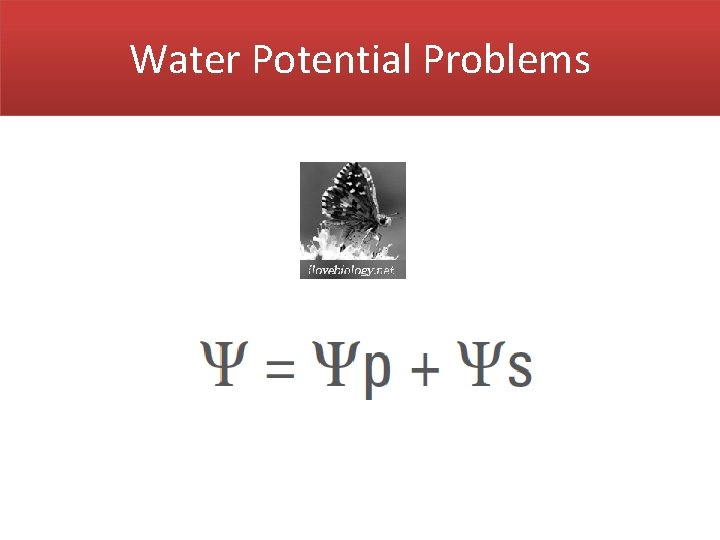Water Potential ProblemsWater Potential ( )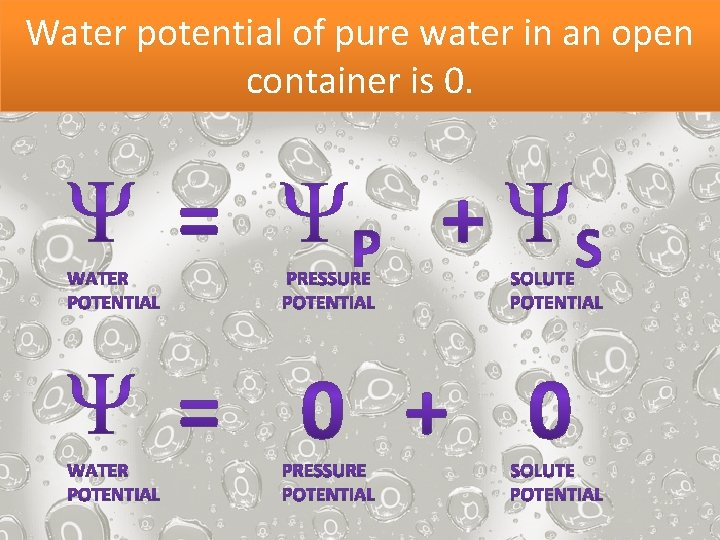Water potential of pure water in an open container is 0.Water will flow from an area of higher water potential to an area of lower water potential.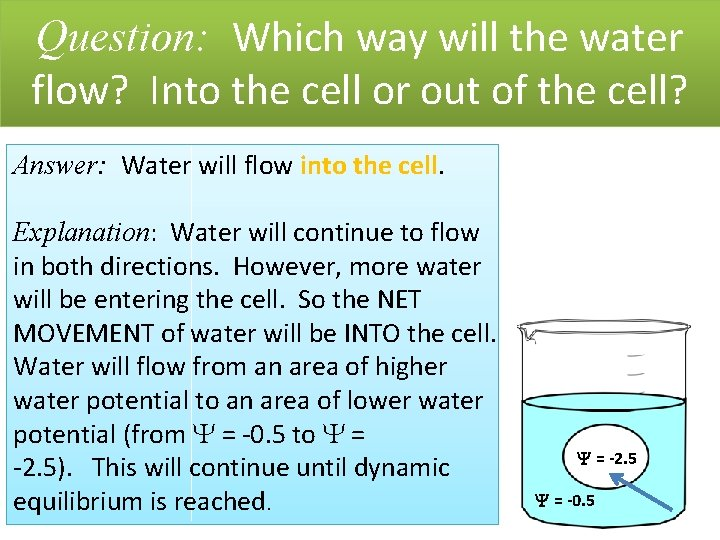Question: Which way will the water flow? Into the cell or out of the cell? Answer: Water will flow into the cell. Explanation: Water will continue to flow in both directions. However, more water will be entering the cell. So the NET MOVEMENT of water will be INTO the cell. Water will flow from an area of higher water potential to an area of lower water potential (from = -0. 5 to = -2. 5). This will continue until dynamic equilibrium is reached. = -2. 5 = -0. 5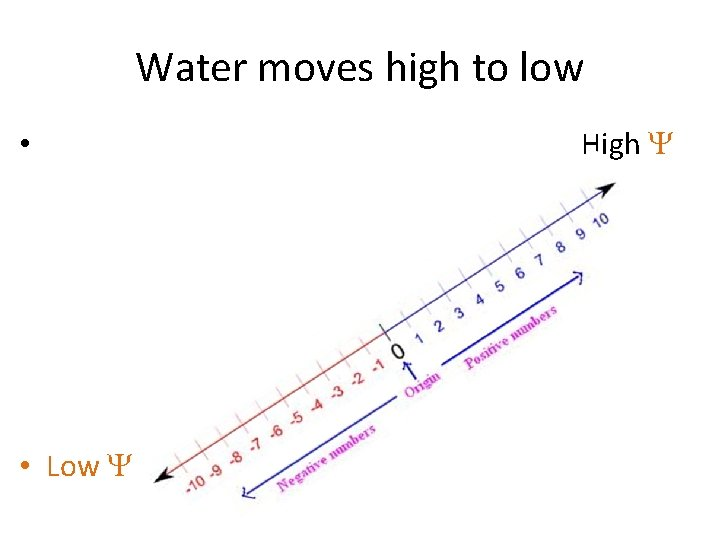Water moves high to low • • Low High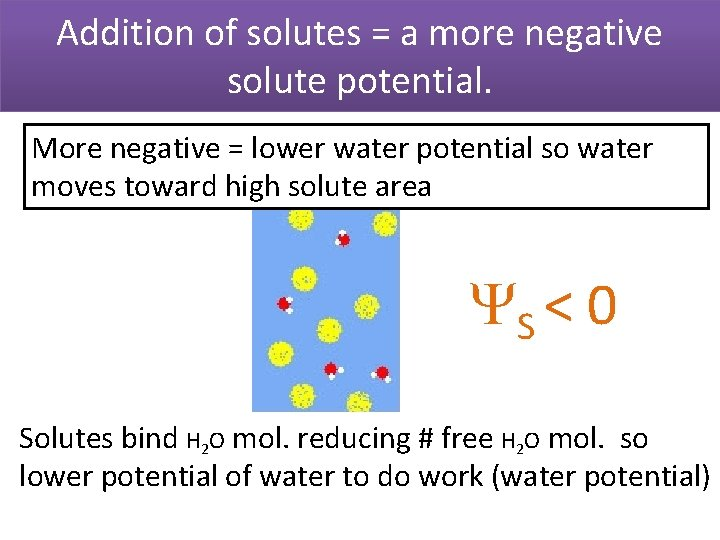Addition of solutes = a more negative solute potential. More negative = lower water potential so water moves toward high solute area S < 0 Solutes bind H 2 O mol. reducing # free H 2 O mol. so lower potential of water to do work (water potential)In an open container or in an animal cell, the pressure potential will be 0. No effect on water movement P = 0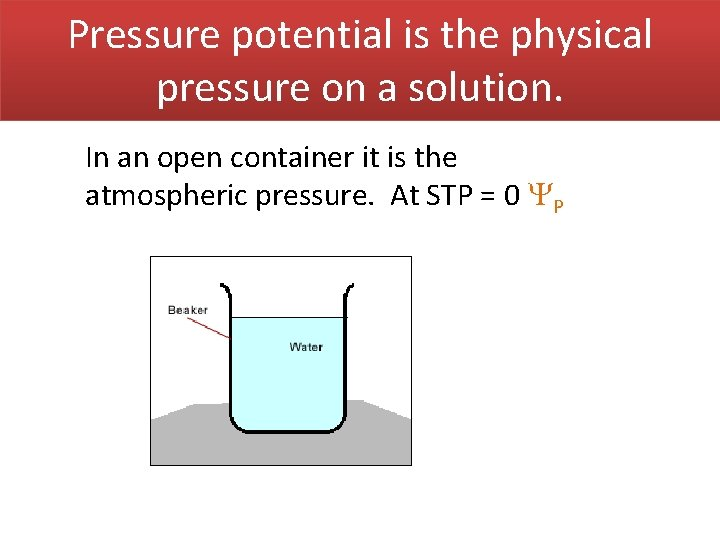Pressure potential is the physical pressure on a solution. In an open container it is the atmospheric pressure. At STP = 0 P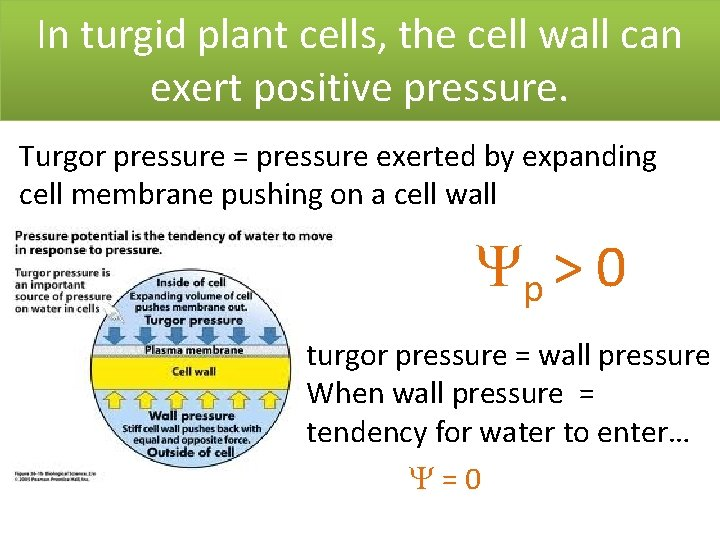In turgid plant cells, the cell wall can exert positive pressure. Turgor pressure = pressure exerted by expanding cell membrane pushing on a cell wall p > 0 turgor pressure = wall pressure When wall pressure = tendency for water to enter… =0Solute Potential ( S)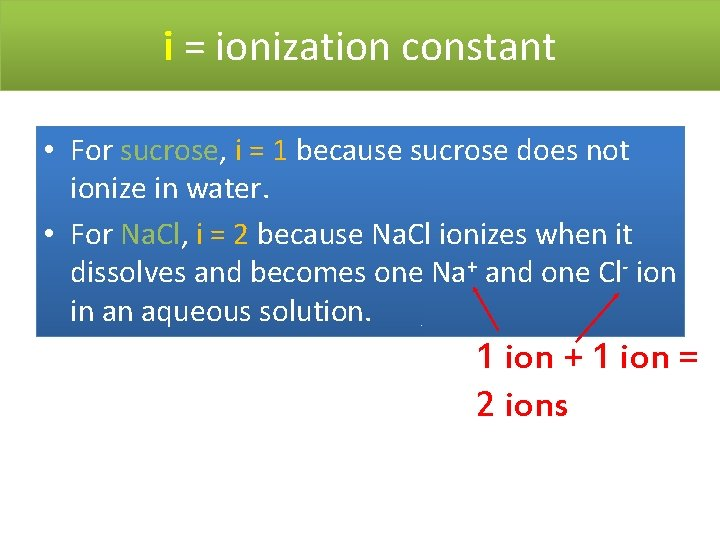i = ionization constant • For sucrose, i = 1 because sucrose does not ionize in water. • For Na. Cl, i = 2 because Na. Cl ionizes when it dissolves and becomes one Na+ and one Cl- ion in an aqueous solution. 1 ion + 1 ion = 2 ions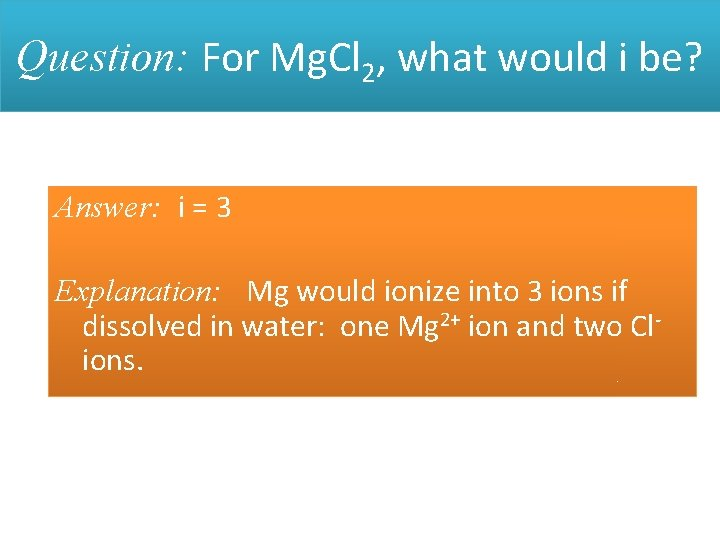Question: For Mg. Cl 2, what would i be? Answer: i = 3 Explanation: Mg would ionize into 3 ions if dissolved in water: one Mg 2+ ion and two Clions.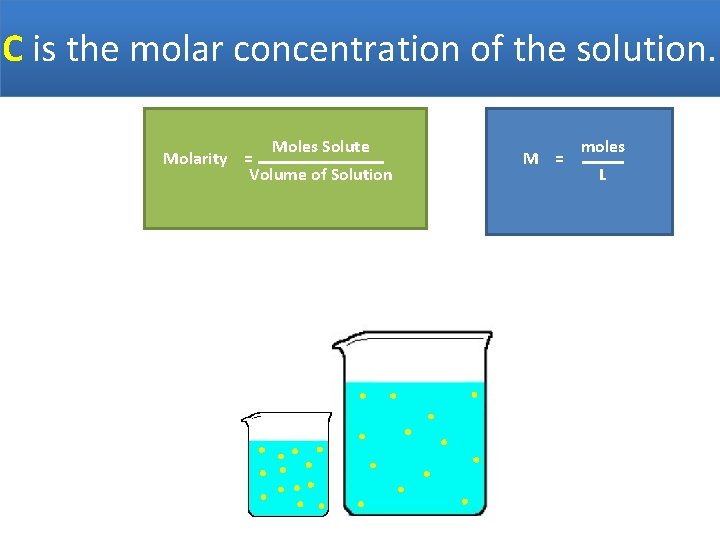C is the molar concentration of the solution. Moles Solute Molarity = Volume of Solution M = moles L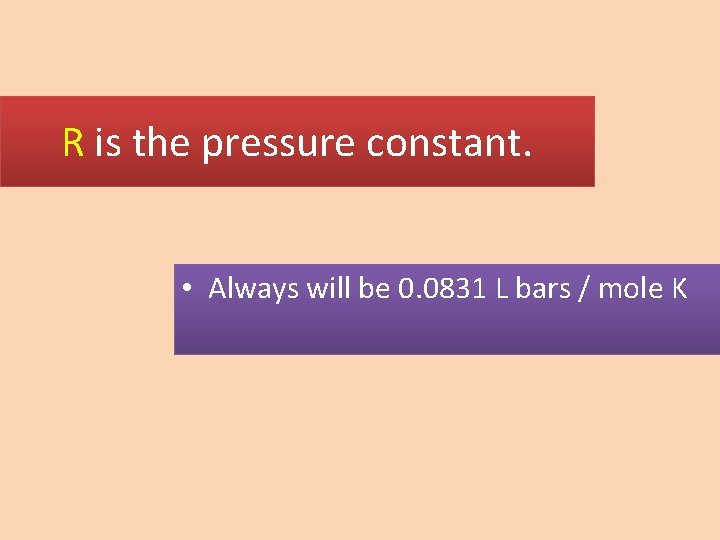R is the pressure constant. • Always will be 0. 0831 L bars / mole K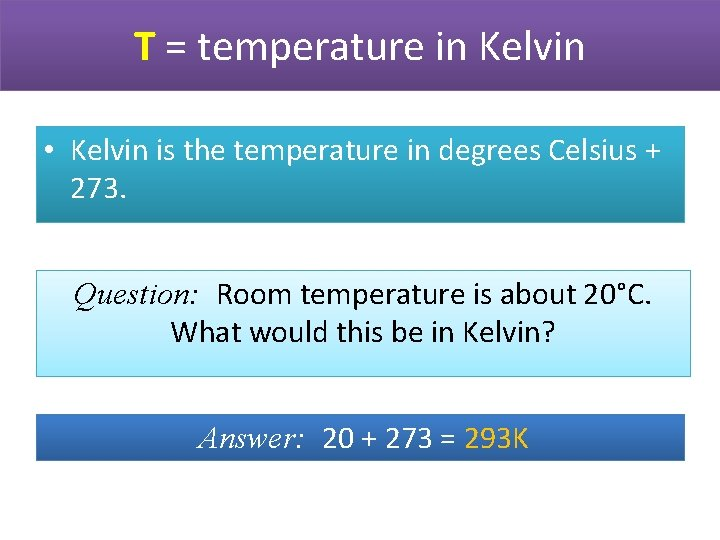T = temperature in Kelvin • Kelvin is the temperature in degrees Celsius + 273. Question: Room temperature is about 20°C. What would this be in Kelvin? Answer: 20 + 273 = 293 K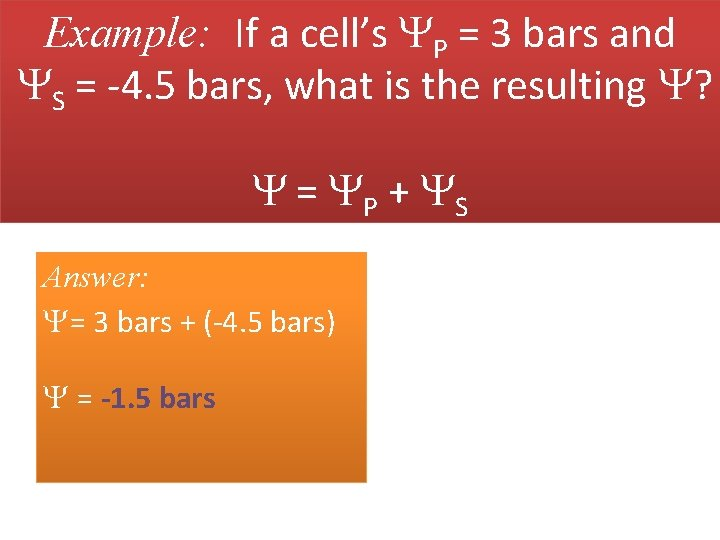Example: If a cell’s P = 3 bars and S = -4. 5 bars, what is the resulting ? = P + S Answer: = 3 bars + (-4. 5 bars) = -1. 5 barsQuestion: A cell with a = -1. 5 bars is placed in a beaker with a solution of = -4 bars. Will water flow into or out of the cell? Answer: Water will flow out of the cell from an area of higher water potential (-1. 5 bars) to an area of lower water potential (-4 bars).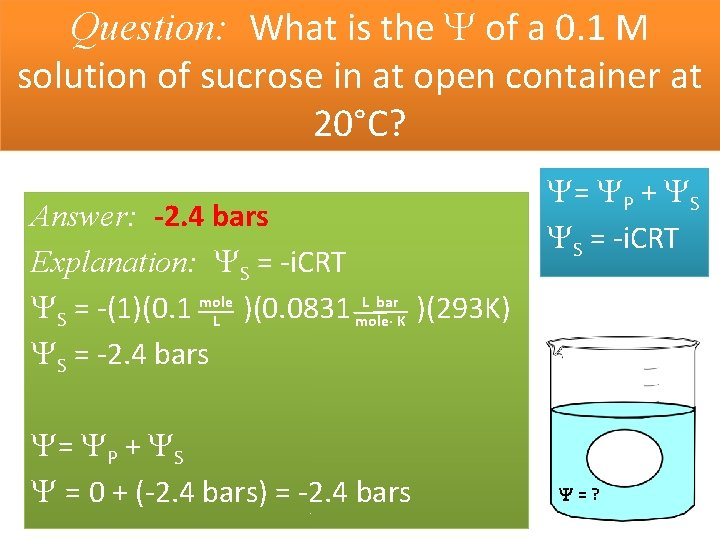Question: What is the of a 0. 1 M solution of sucrose in at open container at 20°C? Answer: -2. 4 bars Explanation: S = -i. CRT L bar S = -(1)(0. 1 mole )(0. 0831 )(293 K) L mole· K S = -2. 4 bars = P + S = 0 + (-2. 4 bars) = -2. 4 bars = P + S S = -i. CRT =?